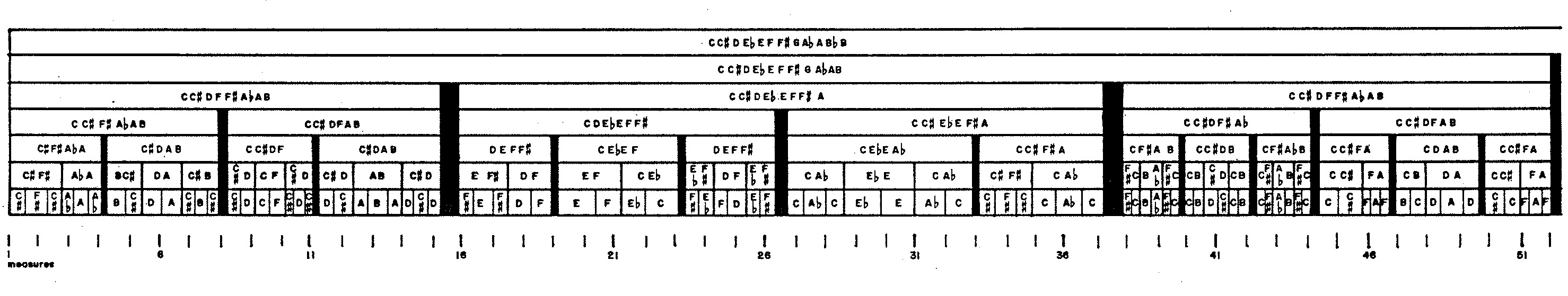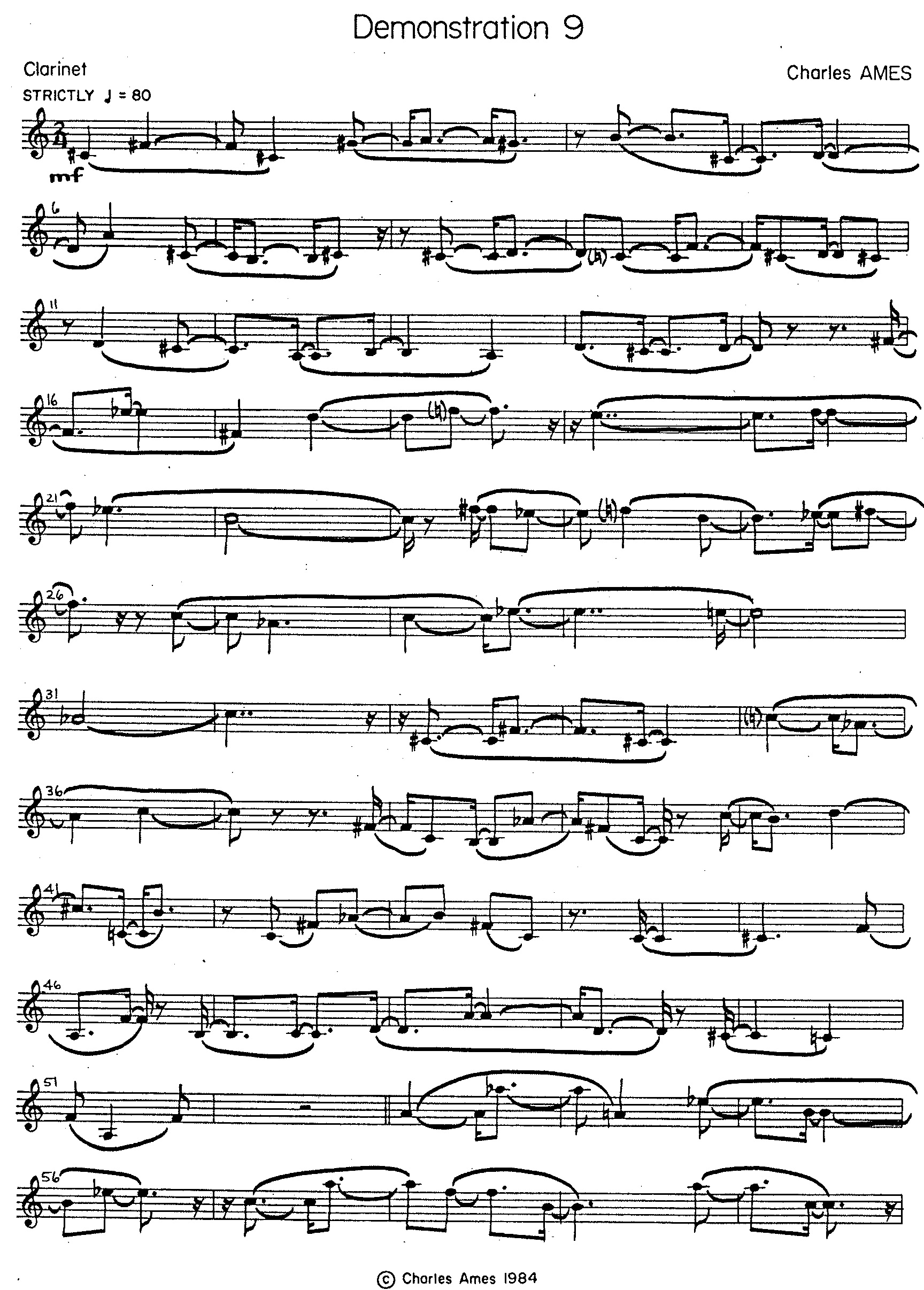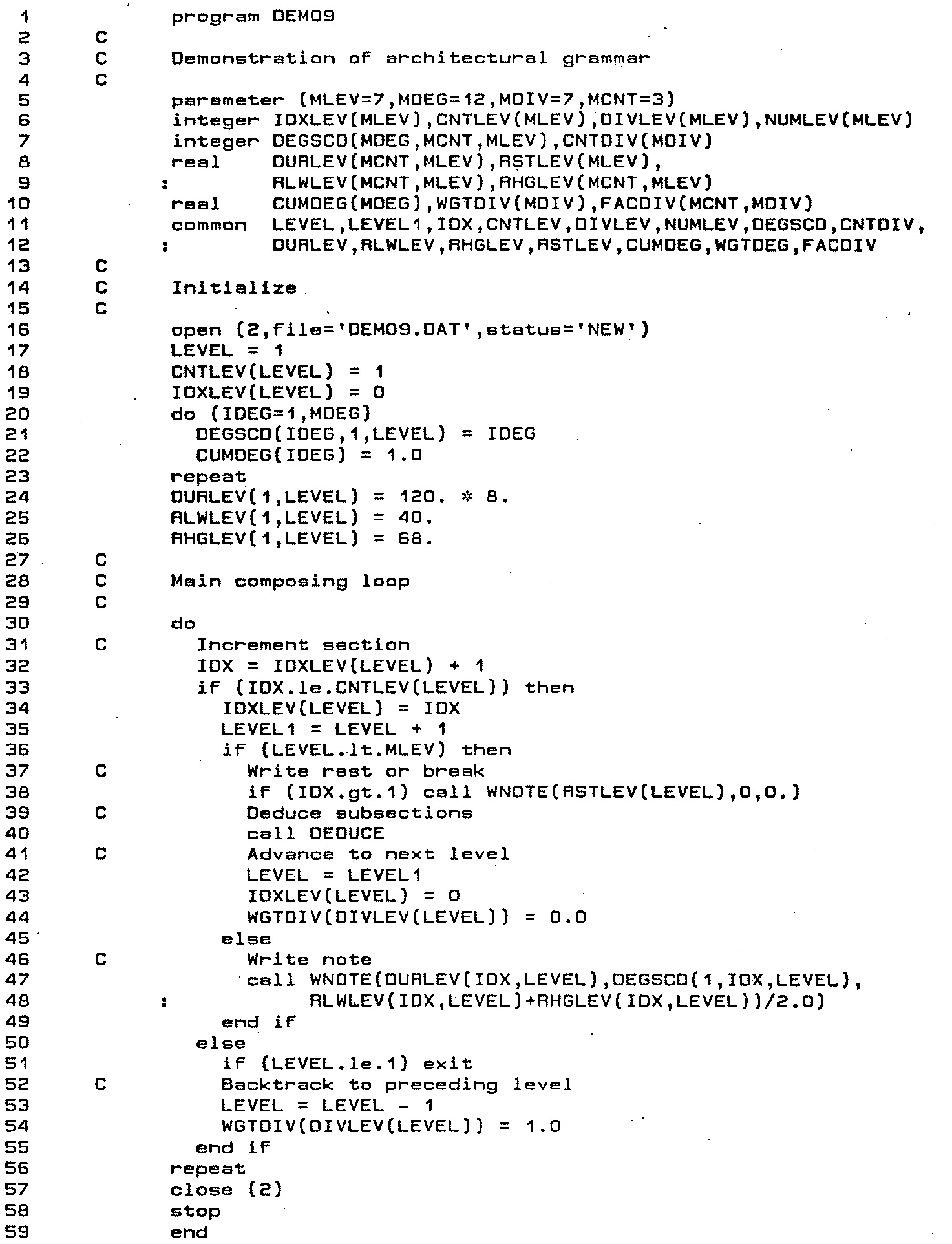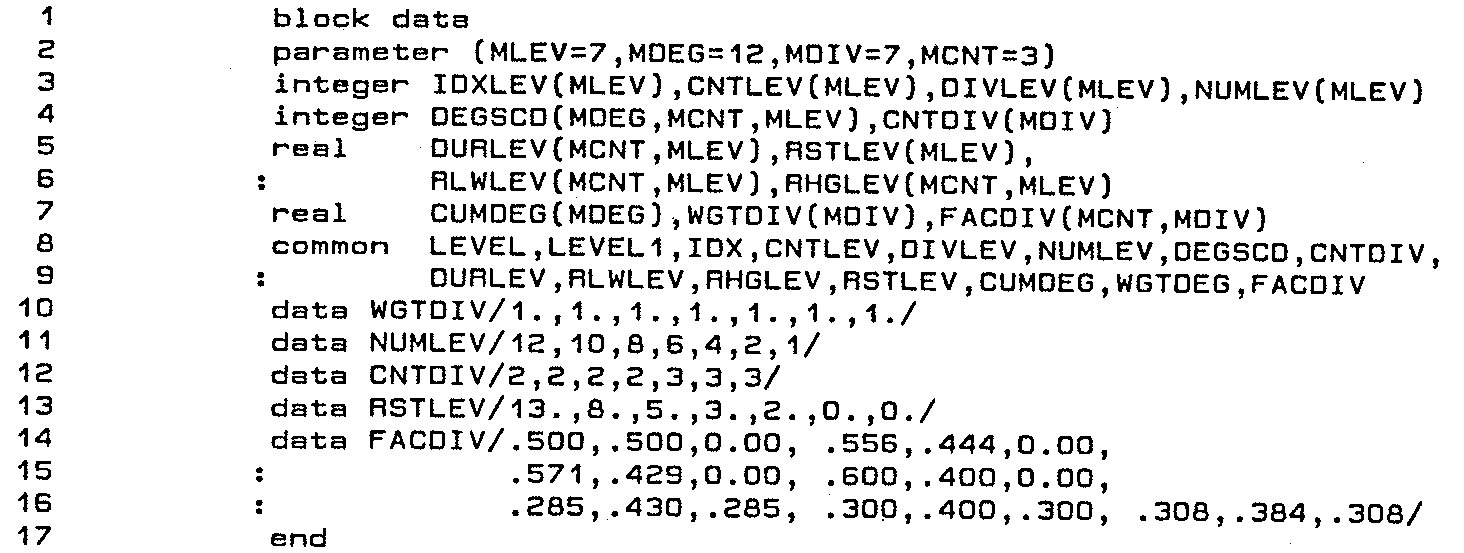# Demonstration 9Architectural Grammar

### Introduction

Demonstration 9 provided the second of two practical components for Chapter 11: “Musical Design II: — Grammars” of my unpublished textbook on composing programs. Like Demonstration 8, Demonstration 9 employs the method of generative grammars and also illustrates the principle of top-down design. This time, the productions divide regions of musical space into nested sub-regions.

### Compositional Directives

The archetype for Demonstration 9 is described by extents along three axes.

1. The duration of the composition (2 minutes),
2. the range of the clarinet, and
3. The twelve degrees of the chromatic scale.Figure 1: Profile of Demonstration 9, measures 1-51.

The productions for Demonstration 9 progressively refine this description by dividing sections into sub-sections and then recursively treating each sub-section as a section in its own right. Figure 1 illustrates this process for measures 1-51 of Demonstration 9. Of the two kinds of production,

• Binary subsections are asymmetric; `A → a b`
• Ternary subsections are symmetric; `A → a b a`

There are four binary modes and three ternary ones; each specific mode is distinguished by the proportions between subsection durations.

In order to project the grammatical structure clearly, no subsection exploits any registral or chromatic resource not already inherent in the section which produced it. To emphasize similarities between the two outer (a) subsections in ternary divisions, ternary productions pass identical resources to outer subsections. To emphasize differences between (a) and (b) subsections in both binary and ternary divisions, productions pass complementary resources to (a) and (b) subsections.

Each act of division deals with registers by dividing the section's extent into two overlapping subranges: a lower range covering the bottom two-thirds of the section extent and an upper range covering the top two thirds of the section extent. Which subrange goes to the (a) subsection is determined by a coin toss, the remaining subrange goes to the (b) subsection.

With regard to chromatic resources, each act of division derives two distinct (though overlapping) subsets from the set of chromatic degrees characterizing the section.

The transcribed product appears in Figure 2 (a) and Figure 2 (b).Figure 2 (a): Figure 2: Transcription of Demonstration 9, page 1. Figure 2 (b): Figure 2: Transcription of Demonstration 9, page 2.

### Implementation

The following explanations seek to illustrate recursive grammars in play. The explanations are peppered with line numbers, but you are are by no means expected to chase down every line of code. Rather, you should follow through with line numbers only when you have a specific question that the narrative is not answering.

Program `DEMO9` proper appears as Figure 3 (a); the `BLOCK DATA` routine used to initialize `COMMON` memory variables appears as Listing 2.Figure 3 (a): Program `DEMO9`. Listing 2: `BLOCK DATA` routine for `DEMO9`.

#### Recursion

Since Demonstration 9 elaborates only one statement of its archetype, the main composing loop in program `DEMO9` proper implements recursive processing. Variable names will be familiar from Demonstration 8's subroutine `COMPOS`: `LEVEL` tracks the level of recursion, iterating from the top down and back. `IDX` tracks horizontal progress, iterating from left to right in the time dimension. Each iteration of `DEMO9`'s main loop (lines 23-49) begins by incrementing `IDX` (line 25), then testing if `IDX` has reached the limit stored in array element `CNTLEV(LEVEL)`. The value of `IDX` is saved (“pushed”) into array element `IDXLEV(LEVEL)` before `LEVEL` advances; `IDX` is retrieved (“popped”) from `IDXLEV(LEVEL)` after `LEVEL` retreats.

For the present purpose, `IDX` identifies a subsection either of the piece as a whole (the archetype) or of a section at the previous recursive level. Sectional attributes are stored in multidimensional arrays indexed both by `IDX` and by `LEVEL`:

• Array element `DURLEV(IDX,LEVEL)` holds the duration of subsection `IDX`.
• Array elements `RLWLEV(IDX,LEVEL)` and `RHGLEV(IDX,LEVEL)` hold lower and upper boundaries, respectively, for a value which will ultimately define the lowest pitch in a twelve-semitone gamut.
• Array element `NUMLEV(LEVEL)` indicates how many degrees of the chromatic scale are to be exploited at the current level. The specific degrees exploited in subsection `IDX` reside in array elements `DEGSCD(1,IDX,LEVEL)` through `DEGSCD(NUMLEV(LEVEL),IDX,LEVEL)`.

Lines 20-26 of program `DEMO09` express the archetype by setting `CNTLEV(1,1)` to 1, `DURLEV(1,1)` to the duration of the composition, `RLWLEV(1,1)` and `RHGLEV(1,1)` to values reflecting the range of the clarinet, NUMLEV(1) to 12, and `DEGSCD(1,1,1)` through `DEGSCD(12,1,1)` to the twelve degrees of the chromatic scale.

For `LEVEL=2` through `LEVEL=6`, line 38 of `DEMO09` articulates consecutive sections with a rest whose duration resides in array element `RSTLEV(LEVEL)`. Since the values of array `RSTLEV` are static, they are populated in line 13 of the `BLOCK DATA` routine.

Remember that I termed Demonstration 9 an “architectural” grammar because notes are generated only at the bottommost level of recursion. Note-generating requests are implemented as calls to subroutine `WNOTE` (lines 47-48).

#### Productions

Productions for Demonstration 9 divide sections into subsections. Implementing these productions boils down to populating values into `DURLEV`, `RLWLEV`, `RHGLEV`, `NUMLEV`, and `DEGSCD` for `LEVEL+1` given values previously populated into these same arrays for `LEVEL`. The code module responsible for this is subroutine `DEDUCE`, which appears in Listing 3 (a) and Listing 3 (b).Listing 3 (a): Subroutine `DEDUCE`, lines 1-71. Listing 3 (b): Subroutine `DEDUCE`, lines 72-138.

The first step in dividing a section into subsections is to choose a mode of division. `DEDUCE` implements this choice in lines 15-29, which places the selected mode in the variable `IDIV`. There four binary modes and three ternary modes. The number of sections for mode `IDIV` is given by array element `CNTDIV(IDIV)` while the duration of subsection `IDX` is given by array element `FACDIV(IDX,IDIV)`. Since the contents of `CNTDIV` and `FACDIV` are static, both arrays are initialized in the `BLOCK DATA` routine. Subsection durations are expressed relative to parent section durations; that is why the values of `FACDIV` sum to unity for each mode of division.

The `BLOCK DATA` routine initializes all elements of array `WGTDIV` to unity, but this array is not static. Here is the mechanism which prevents any one mode of division from being chosen more than once along any vertical path one might trace down through the structure. Array element `WGTDIV(IDIV)` holds the relative likelihood of selecting mode `IDIV`. For `LEVEL=1`, each element of `WGTDIV` has its initialized value of unity. Thus all modes have equal likelihood of selection. Should the choice for `IDIV` at `LEVEL=`L be I, then I is populated into array element `DIVLEV(`L`)` while `WGTDIV(`I`)` is cleared to zero (line 44 of program `DEMO09`). This prevents mode I from being reselected at any lower level. When the composing process retreats back to `LEVEL=`L, array element `WGTDIV(`I`)` resets to unity (line 54 of `DEMO09`).

Line 21 of subroutine `DEDUCE` dereferences the subsection count from array element `CNTDIV(IDIV)` into variable `LCNT`. The mechanism for selecting durations of subsections varies with LCNT as follows:

• If the mode of division is binary, then lines 31-35 apply. `DEDUCE` selects the variable `MORE` to indicate the longer subsection while selecting the variable `LESS` to indicate the shorter subsection. Understand that my library function `IRND` called in line 32 generates random numbers ranging from 1 up to the indicated argument. Thus `DEDUCE` selects either `MORE=1` and `LESS=2` or `MORE=2` and `LESS=1` with equal likelihood. Lines 34-35 dereference relative proportions from `FACDIV(1,IDIV)` and `FACDIV(2,IDIV)`, scale these proportions to the section duration, then distribute the results to subsections.
• If the mode of division is ternary, then lines 38-42 apply. The middle subsection is always the longest. Array elements `FACDIV(1,IDIV)`, `FACDIV(2,IDIV)`, and `FACDIV(3,IDIV)` holds the relative proportions which lines 40-42 scale to the section duration, then distribute to subsections.

Lines 47-71 of subroutine `DEDUCE` compute subsection ranges as two-thirds of the section extent, positioned either at the bottom or at the top. The library subroutine `SUCCES(P)` implements a Bernoulli trial with output values 0 (probability P) or 1 (probability 1-P). Having the probability set to 1/2 in line 48 means that which range (lower or upper) goes with which subsection is decided by a coin toss.

Lines 76-136 of subroutine `DEDUCE` allocates chromatic degrees to subsections. This process most heavily favors those degrees which have been least exploited elsewhere in the piece.

The first step uses the library subroutine `DSORT` to marshal the current section's chromatic set into a preference order. `DSORT` expects an integer array for argument 1, a real array for argument 2, and a simple integer for argument 3. The integer array contains the values which will be sorted into descending order based on preference values stored in the real array. Argument 3 indicates how many values to sort. Remember that FORTRAN passes subroutine arguments by address rather than by value, so that what `DSORT` does to reorder the integer array remains valid in the calling routine.

Array element `CUMDEG(`I`)` holds the total duration (in sixteenth notes) of all sections in which degree I has been eliminated up to the current point in the composing process. Line 82 sorts the current section's chromatic set into a preference order; however, using `CUMDEG` values directly for this purpose means that if two degrees share the same `CUMDEG` value, then the sorting algorithm will favor the degree with the lower chromatic number. To eliminate such favoritism, each `CUMDEG` value receives an increment generated randomly between zero and unity. Lines 77-81 store these adjusted sums in array `FUZDEG`.

The expression `call DSORT(DEGSCD(1,IDX,LEVEL),FUZDEG,NUM)` translates into English as follows: sort `NUM` successive integers starting with the address of `DEGSCD(1,IDX,LEVEL)` into descending order based on the preference values stored in array `FUZDEG`. We know `DSORT` will receive the right content by understanding that FORTRAN stores the content of the multidimensional `DEGSCD` array as a contiguous block of `MDEG`×`MCNT`×`MLEV` integers, arranged in the following sequence:

 `DEGSCD(1,1,1)` `DEGSCD(2,1,1)` … `DEGSCD(MDEG,1,1)` `DEGSCD(1,2,1)` `DEGSCD(2,2,1)` … `DEGSCD(MDEG,2,1)` ⋮ ⋮ ⋮ ⋮ `DEGSCD(1,MCNT,1)` `DEGSCD(2,MCNT,1)` … `DEGSCD(MDEG,MCNT,1)` ⋮ ⋮ ⋮ ⋮ `DEGSCD(1,MCNT,MLEV)` `DEGSCD(2,MCNT,MLEV)` … `DEGSCD(MDEG,MCNT,MLEV)`

How subroutine `DEDUCE` populates chromatic degrees into array `DEGSCD` for `LEVEL+1` depend both upon the recursive level and the mode of division. Whenever a degree is excluded from a subsection, the corresponding `CUMDEG` element increases by the subsection's duration.

• For `LEVEL=1` through `LEVEL=5`, all degrees in the schedule except for the last four are passed to all subsections. The remaining four degrees are dereferenced into variables `IDEG1`, `IDEG2`, `IDEG3`, and `IDEG4` in reverse1 order of preference.
• If the mode of division is binary, then lines 97-102 apply. `DEDUCE` passes `IDEG1` and `IDEG2` to the longer subsection while passing `IDEG3` and `IDEG4` to the shorter subsection.
• If the mode of division is ternary, then lines 104-111 apply. `DEDUCE` passes `IDEG1` and `IDEG2` to the outer (a) subsections while passing `IDEG3` and `IDEG4` to the inner (b) subsection. Notice that the combined durations of (a) subsections is consistently at least as large as the duration of the (b) subsection.
Lines 114-117 increase the `CUMDEG` values for `IDEG1`, `IDEG2`, `IDEG3`, and `IDEG4` by the durations of the subsections where these degrees were not placed.
• The two degrees remaining at level 6 are handled similarly:
• If the mode of division is binary, then lines 124-127 apply. `DEDUCE` dereferences them into variables `IDEG1` and `IDEG2`, in direct order of preference. `IDEG1` passes down to the longer subsection, while `IDEG2` passes down to the shorter subsection. Lines 126-127 increase the `CUMDEG` values for `IDEG1` and `IDEG2` as appropriate.
• If the mode of division is ternary, then lines 129-134 apply. `IDEG1` passes down to the inner (a) subsection, while `IDEG2` passes down to the outer (b) subsection. Lines 132-134 increase the `CUMDEG` values for `IDEG1` and `IDEG2` as appropriate.

As a result of this process, local chromatic distributions are very much non-uniform. However if you sum together the durations for each chromatic degree over the composition as a whole, the sums should be pretty much even.